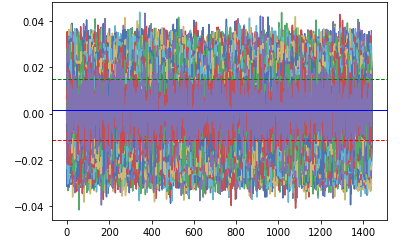# Managing Portfolio Risk Using Monte Carlo Simulations

##### Calculating Value at Risk (VaR) to manage financial risk of a portfolio using Monte Carlo Simulations in Python
###### VaR in Financial and Portfolio Risk Management?

VaR is an acronym of ‘Value at Risk’, and is a tool which is used by many firms and banks to establish the level of financial risk within its firm. The VaR is calculated for an investments of a company’s investments or perhaps for checking the riks levels of a portfolio managed by the wealth management branch of a bank or a boutique firm.

The calculation may be thought of as a statistical measure in isolation. It can also be simplified to the following example statement –

VaR is the minimum loss which will be incurred at a certain level of probability (confidence interval) OR the maximum loss which will be realized at a level of probability.

The above image shows the maximum loss which can be faced by a company at a α% confidence. On a personal level VaR can help you predict or analyse the maximum losses which your portfolio is likely to face — this is something which we will analyse soon.

###### Monte Carlo Simulations

The Monte Carlo model was the brainchild of Stanislaw Ulam and John Neumann, who developed the model after the second world war. The model is named after a gambling city in Monaco, due to the chance and random encounters faced in gambling.

The Monte Carlo simulation is a probability model which generates random variables used in tandem with economic factors (expected return, volatility — in the case of a portfolio of funds) to predict outcomes over a large spectrum. While not the most accurate, the model is often used to calculate the risk and uncertainty.

We will now use the Monte Carlo simulation to generate a set of predicted returns for our portfolio of assets which will help us to find out the VaR of our investments.

###### Calculating VaR in Python

We will first set up the notebook by importing the required libraries and functions

`#Importing all required libraries#Created by Sanket Karveimport matplotlib.pyplot as pltimport numpy as npimport pandas as pdimport pandas_datareader as webfrom matplotlib.ticker import FuncFormatter!pip install PyPortfolioOpt#Installing the Portfolio Optimzation Library`
`from pypfopt.efficient_frontier import EfficientFrontierfrom pypfopt import risk_modelsfrom pypfopt import expected_returnsfrom matplotlib.ticker import FuncFormatter`

For the purposes of our project, I have considered the ‘FAANG’ stocks for the last two years.

`tickers = ['GOOGL','FB','AAPL','NFLX','AMZN']thelen = len(tickers)price_data = []for ticker in range(thelen):`
`   prices = web.DataReader(tickers[ticker], start='2018-06-20', end = '2020-06-20', data_source='yahoo')`
`   price_data.append(prices[['Adj Close']])`
`df_stocks = pd.concat(price_data, axis=1)df_stocks.columns=tickersdf_stocks.tail()`

For the next step, we will calculated the portfolio weights of each asset. I have done this by using the asset weights calculated for achieving the maximum Sharpe Ratio. I have posted the snippets of the code for the calculation below.

`#Annualized Returnmu = expected_returns.mean_historical_return(df_stocks)#Sample Variance of PortfolioSigma = risk_models.sample_cov(df_stocks)`
`#Max Sharpe Ratio - Tangent to the EFfrom pypfopt import objective_functions, base_optimizeref = EfficientFrontier(mu, Sigma, weight_bounds=(0,1)) #weight bounds in negative allows shorting of stockssharpe_pfolio=ef.max_sharpe() `
`#May use add objective to ensure minimum zero weighting to individual stockssharpe_pwt=ef.clean_weights()print(sharpe_pwt)`

The asset weights will be used to calculate the expected portfolio return.

`#VaR Calculationticker_rx2 = []`
`#Convert Dictionary to list of asset weights from Max Sharpe Ratio Portfolio`
`sh_wt = list(sharpe_pwt.values())sh_wt=np.array(sh_wt)`

Now, we will convert the stock prices of the portfolio to a cumulative return, which may also be considered as the holding period returns (HPR)for this project.

`for a in range(thelen):`
`  ticker_rx = df_stocks[[tickers[a]]].pct_change()  ticker_rx = (ticker_rx+1).cumprod()  ticker_rx2.append(ticker_rx[[tickers[a]]])`
`ticker_final = pd.concat(ticker_rx2,axis=1)ticker_final`
`#Plot graph of Cumulative/HPR of all stocks`
`for i, col in enumerate(ticker_final.columns):  ticker_final[col].plot()`
`plt.title('Cumulative Returns')plt.xticks(rotation=80)plt.legend(ticker_final.columns)`
`#Saving the graph into a JPG fileplt.savefig('CR.png', bbox_inches='tight')`

Now, we will pick out the latest HPR of each asset and multiply the returns with the calculated asset weights using the .dot() function.

`#Taking Latest Values of Return`
`pret = []pre1 = []price =[]`
`for x in range(thelen):  pret.append(ticker_final.iloc[[-1],[x]])  price.append((df_stocks.iloc[[-1],[x]]))`
`pre1 = pd.concat(pret,axis=1)pre1 = np.array(pre1)price = pd.concat(price,axis=1)varsigma = pre1.std()ex_rtn=pre1.dot(sh_wt)`
`print('The weighted expected portfolio return for selected time period is'+ str(ex_rtn))`
`#ex_rtn = (ex_rtn)**0.5-(1) #Annualizing the cumulative return (will not affect outcome)`
`price=price.dot(sh_wt) #Calculating weighted valueprint(ex_rtn, varsigma,price)`

Having calculated the expected portfolio return and the volatility (standard deviation of the expected returns), we will set up and run the Monte Carlo simulation. I have used a time of 1440 (no of minutes in a day) with 10,000 simulation runs. The time-steps may be changed per requirement. I have used a 95% confidence interval.

`from scipy.stats import norm`
`import math`
`Time=1440 #No of days(steps or trading days in this case)`
`lt_price=[]`
`final_res=[]`
`for i in range(10000): #10000 runs of simulation`
`  daily_return=                     (np.random.normal(ex_rtn/Time,varsigma/math.sqrt(Time),Time))  plt.plot(daily_returns)`
`plt.axhline(np.percentile(daily_returns,5), color='r', linestyle='dashed', linewidth=1)`
`plt.axhline(np.percentile(daily_returns,95), color='g', linestyle='dashed', linewidth=1)`
`plt.axhline(np.mean(daily_returns), color='b', linestyle='solid', linewidth=1)`
`plt.show()`Range of returns in a day of 1440 minutes | Red dash — Min Loss | Green Dash — Min Gain

Visualizing the distribution plot of the returns presents us with the following chart

`plt.hist(daily_returns,bins=15)`
`plt.axvline(np.percentile(daily_returns,5), color='r', linestyle='dashed', linewidth=2)`
`plt.axvline(np.percentile(daily_returns,95), color='r', linestyle='dashed', linewidth=2)`
`plt.show()`

Printing the exact values at both the upper limit and lower limit and assuming our portfolio value to be \$1000, we will calculated an estimate of the amount of funds which should be kept to cover for our minimum losses.

`print(np.percentile(daily_returns,5),np.percentile(daily_returns,95)) #VaR - Minimum loss of 5.7% at a 5% probability, also a gain can be higher than 15% with a 5 % probability`
`pvalue = 1000 #portfolio value`
`print('\$Amount required to cover minimum losses for one day is ' + str(pvalue* - np.percentile(daily_returns,5)))`